# Tower Of Hanoi CodeChef Solution 2022Hello geeks 😉, in this article we are going to discuss about the Tower Of Hanoi problem which is a very famous mathematical puzzle. We will be discussing :

• Brief story about the problem to understand it better
• Approach to solve this problem
• Time complexity
• Space complexity
Contents

# Story

There are some stories associated with Tower of Hanoi but here we will cover only one. It is said that there is temple of lord brahma in south India where there are three towers, one of the tower has 64 golden disks on it. Tower of Hanoi puzzle is also know as tower of brahma puzzle. It is said that when brahma created the universe he gave a task to some priests to keep all the disks from first tower to the third tower.

Brahma also gave some simple rules/conditions to the priests that they have to keep the plates in ascending order from top to bottom i.e., the smallest disk at the top and the largest disk at the bottom, no larger disk can be placed on top of smaller disk. Also at one time they can pick only one disk and use the second tower as a help to move the disk. It is also said that if the task is completed the universe will end and brahma will start the new cycle of universe.

# Problem statement

Consider 3 pegs, peg A, peg B and peg C. There are n disks are on peg A, objective of the puzzle is to move the stack of disks to peg C, using peg B(if needed) such that

• No larger disk is kept over a smaller disk
• Only one disk is moved at a time
• Only top disc of a peg is moved

The required output will be the steps to be followed so that the above puzzle is solved like move disk 1 to peg B.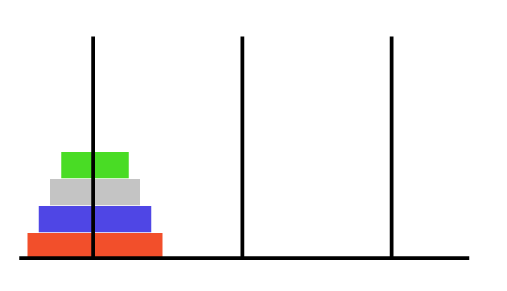Peg A                      Peg B                  Peg C

# How to Approach ?

To solve the above problem we will be using mathematical induction and recursion.

Consider we have 1 disk on peg A and we are required to move it. We can simply move disk1 from peg A to peg C.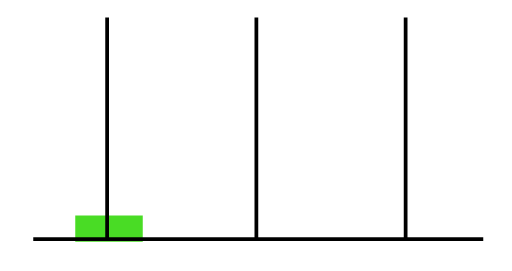Peg A              Peg B                  Peg C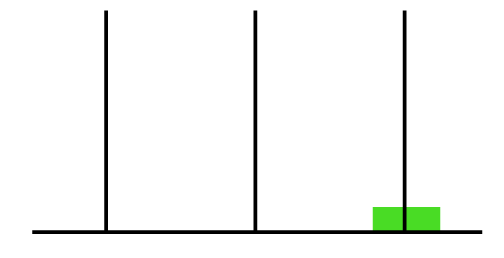Peg A                  Peg B                 Peg C

Now let us consider that we have 2 disks. This can be solved simply, we will move disk 1 to peg B (auxiliary) then disk 2 to peg C then finally again disk 1 to peg C. So following this step we can move two disks from one peg to another.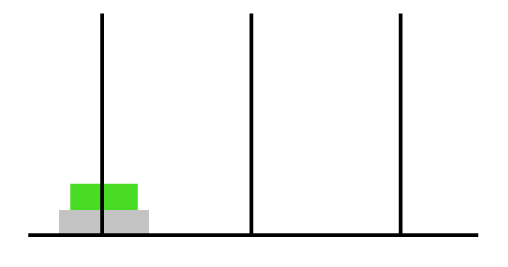Peg A                      Peg B                  Peg C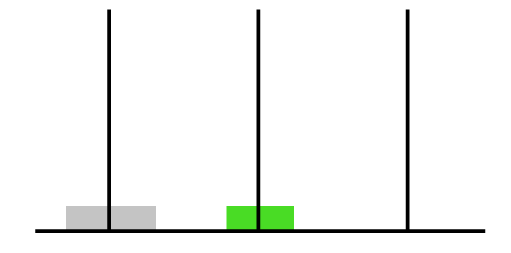Peg A                      Peg B                  Peg C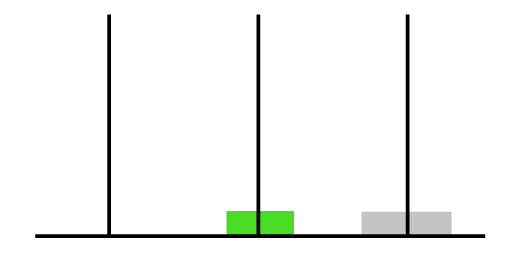Peg A                      Peg B                  Peg C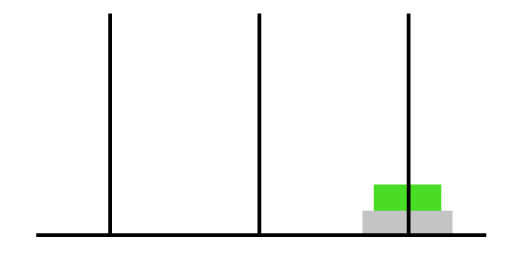Peg A                      Peg B                  Peg C

Now consider we have 3 disks. To solve we will first move top 2 disks to peg B using the same logic as we have discussed above (Just in this case auxiliary will be C). Then we will move disk 3 to peg C . Now we will again follow same steps to move the two disks from peg B to peg C.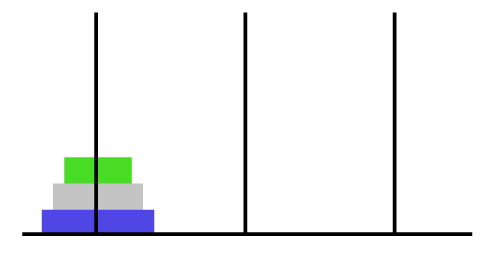Peg A                      Peg B                  Peg C

Now if we look there exists a recursion i.e., first we are calling the recursive function to move top n-1 disks from peg A to peg B then we move the nth disk from peg A to peg C. After that we will again call the recursive function to move n-1 disks at peg B to peg A.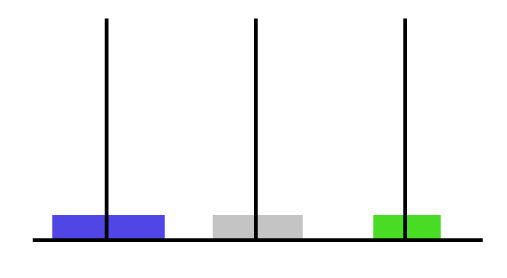Peg A                      Peg B                  Peg C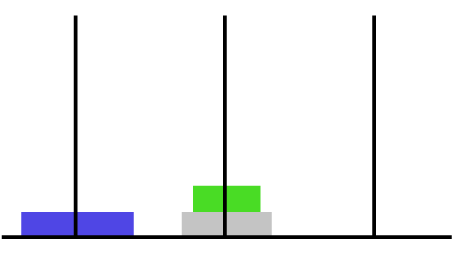Peg A                      Peg B                  Peg C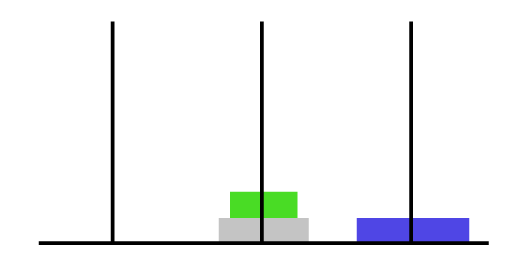Peg A                      Peg B                  Peg C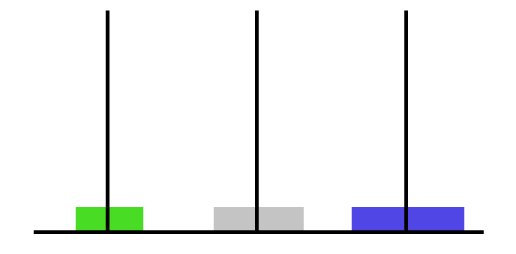Peg A                      Peg B                  Peg C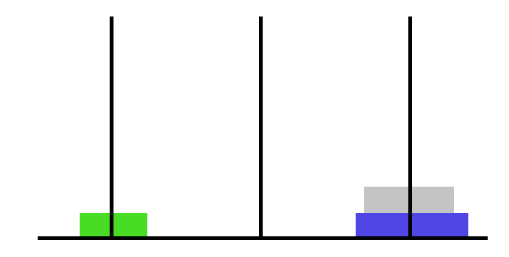Peg A                      Peg B                  Peg C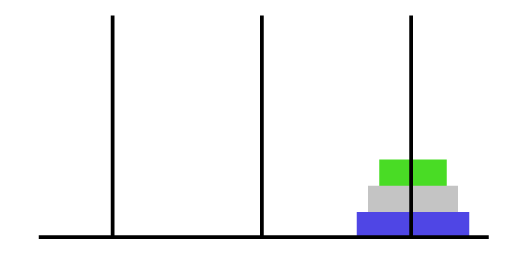Peg A                      Peg B                  Peg C

We are moving n-1 disks two times (first from A to B then from B to C ) and we move the nth disk only once (from A to C ).

So the required recurrence relation is an = 2an-1 +1.

If we want to solve for 4 disks then we will apply similar steps as we did for 3. First we will move n-1 i.e., 3 disks from peg A to peg B recursively . then we will move the nth disk from peg A to peg C . Then we will move the n-1 disks at peg B to peg C recursively.

This will solve the puzzle for 4 disks.

Similar steps can be applied for any number of disks. The minimum number of moves can be deduced from the recurrence relation easily.

Each an can be written as

an = 2*(2*an-2 +1)+1.

which can be further written as an = 2*(2*(2*an-3 +1)+1)+1 similarly we can find for all terms till a1 (whose value we know is 1) so, the

minimum number of moves to move n disks is 2n-1

### C++ code

```#include <iostream>
using namespace std;
// recursive function for tower of hanoi
void towerofhanoi(int n, char source, char aux, char destination)
{
if (n == 0) // base case
{
return;
}
towerofhanoi(n - 1, source, destination, aux); // Call to move n-1 disks from source to aux peg
cout << "Move disk " << n << " From peg " << source << " to peg " << destination << endl;
towerofhanoi(n - 1, aux, source, destination); // Call to move n-1 aux peg to destination peg
}
int main()
{
int n = 4;                     // number of disks
towerofhanoi(n, 'A', 'B', 'C'); // function call
return 0;
}```

### C code

```#include <stdio.h>
// recursive function for tower of hanoi
void towerofhanoi(int n, char source, char aux, char destination)
{
if (n == 0) //base case
{
return;
}
towerofhanoi(n - 1, source, destination, aux); // Call to move n-1 disks from source to aux peg
printf("Move disk %d From peg %c to peg %c \n", n, source, destination);
towerofhanoi(n - 1, aux, source, destination); // Call to move n-1 aux peg to destination peg
}
int main()
{
int n = 4;                      // number of disks
towerofhanoi(n, 'A', 'B', 'C'); // function call
return 0;
}```

### Java code

```class towerofhanoi {
static void tower_of_hanoi(int n, char source, char aux, char destination) {
if (n == 0) //base case
{
return;
}
tower_of_hanoi(n - 1, source, destination, aux); // Call to move n-1 disks from source to aux peg
System.out.println("Move disk " + n + " From peg " + source + " to peg " + destination);
tower_of_hanoi(n - 1, aux, source, destination); // Call to move n-1 aux peg to destination peg
}

public static void main(String[] args) {
int n = 4; // number of disks
tower_of_hanoi(n, 'A', 'B', 'C'); // function call
}
}
```

Time Complexity : Time complexity of the above recursive algorithm is O(2n). For explanation of this you can refer to the above sections.

Also Read : 3SUM (LeetCode Problem)

# FAQ

Q)Why is it so hard to solve the Tower of Hanoi puzzle?

Ans) It is not difficult to solve Tower of Hanoi puzzle. You just need knowledge of recursion and recurrence relation then you can implement the algorithm to solve tower of Hanoi easily.

Q)What is the Tower of Hanoi puzzle?

Ans)Tower of Hanoi puzzle is a mathematical problem which consists of 3 pegs or rods and some number of discs arranged in ascending order from top to bottom in which we are required to move the stack of disks from one peg/rod to other using the third rod/peg as an auxiliary one and also by following some certain conditions.

Q)How do I solve the Tower of Hanoi puzzle?

Ans)Tower of Hanoi puzzle can be solved easily using recursion and recurrence relation. We have to call the recursive function for n-1 disks.

### Conclusion

In this article we learnt how to approach and solve Tower of Hanoi puzzle using recursion and mathematical induction. It can be solved very easily if we understand concept of recursion properly. The crux of the approach was to identify the base case and how to divide other cases into sub-parts.